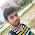### Mathematics for Competitive Programming ( Course )

Hi there, in this course I will be teaching Maths concept used in competitive programming with complete explanation with proofs and implementation in C++. This course is a Lengthy Course as it consists of many subtopics and concepts which we can't skip of fast forward.

This course will be covering Number Theory, Combinatorics, and Computational Geometry. And we will be starting with the first topic Number Theory then we will be going through Combinatorics and then Computational Geometry.

Number Theory: ( Lectures on YouTube)
Practice Problem Set - 1

Note: More Topics will be added as we proceed. Each lecture will be followed by example problem and practice problem link will be shared here itself.

For your suggestion and feedback do comment here.
Thank You
CODELABS3277

1.